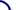Centrale des maths - centraledesmaths.uregina.caCentre de ressources« centre de ressources »VoletSecondaire -Les équations et la résolution de problèmesnouvelle recherche

Nous avons trouvé 34 articles correspondant à votre recherche.Page1/3Dans l'exemple ci-dessous, nous partons d'une situation familière è l'élève pour arriver au modèle mathématique. Un des avantages de cette activité est qu'il n'y a pas de solution unique. Trouver diversesIn this note Gregory describes a problem involving Dasher and Dancer moving around a Northern Light Circle.A diamond slope, or the slope of the angle bisector, is considered in this note as a generalization of two well-known slope relationships. This general approach is compared then with well-known approaches using various examples.Karen designed this website to assist teachers and pre-service teachers in the area of mathematics from Kindergarten to Grade 12 . Here you will find a multitude of teacher resources to assist you in incorporating Aboriginal content in your mathematics program.Against all Odds (HTML ou PDF)This unit was developed for the beginning secondary level and gives students a chance to both learn valuable mathmatics skills and to become aware of the impact gambling has on our society. The unit provides objectives, evalution ideas and suggested activities for students. Also listed are resource materials that can be used with this unit.This resource contains instructions on building a uniform polyhedra "star ball" from modules of folded paper. Animation is used to illustrate the folding of the paper. Students are then challenged to construct other uniform polyhedra from the same modules and to discover how they can be "coloured" by using coloured paper. The construction should be possible for beginning middle year students and some of the questions challenging to students at the upper secondary level.Some main concepts discussed in this Stewart Resource unit are properties of polygons, Pythagorean Theorem and Trionometric Ratios. There are five main sections each with corresponding activities. Activites include sections on Objectives, Background Knowledge, Time frame,Iinstructional Methods, Aadaptive Dimension and Assessment.Gregory and Oleksandr have built on the arc midpoint resource and the proof of the arc midpoint formula by constructing an algorithm for finding the coordinates of the midpoint. It is hoped that teachers of high school Mathematics and Computer Science will use these resources to enrich the teaching and learning in both subject areas.Gregory and Oleksandr use their Arc Midpoint Computation approach to solve a problem concerning gravitational potential energy and then challenge the reader to solve the same problem using an alternative approach.Gregory finds another application of his arc midpoint computation, this time to the kinetic energy of an object moving along a semicircle.Oleksandr and Gregory extend interpretations of non-piecewise identities for sin^(-1)x+ sin^(-1)y and cos^(-1)x+ cos^(-1)y using several Euclidean geometry statements and illustrations.Consumer Wise (HTML ou PDF)This secondary unit helps teach students the importance of being "consumer wise" now and after graduation. Income, Budgeting and Credit, Saving and Loans are a few of the topics discussed in the activities. Worksheets for the activities are included in this unit as well as objectives, evaluation and resources ideas.In this note Gregory uses a trig identity to develop an expression for the slopes of the angle bisectors of two lines in terms of the slopes of the lines that form the angle.A trigonometric identity is used to develop a formula for the slope of a rhombus diagonal. This expression is then used to find the velocity of a whale.A unit on direct and partial variation that can be taught after the introduction of linear functions.Page1/3Centrale des maths reçoit une aide financière de l’Université de Regina et de The Pacific Institute for the Mathematical Sciences.Qui sommes-nous :: carte du site :: our english site